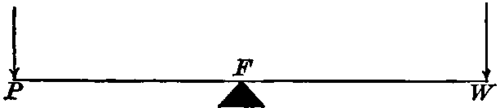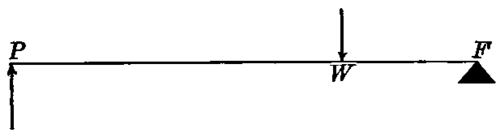In this form (Fig. 36), the fulcrum or fixed supporting point, F, lies between the weight to be moved and the moving power. The distance P F from the power to the fulcrum is called the power-arm of the lever, and the distance WF is the weight-arm. When power-arm and weight-arm are equal (as in an ordinary pair of scales), no mechanical advantage is gained ; to lift a pound at W, P must be pressed down with a force greater than a pound ; and the end W will go up just as far as the end P goes down. If PF be longer than WF then a small weight at P will balance a larger one at W, the gain being greater the greater the difference in the length of the arms, but the distance through which W is moved will be less than that through which P moves ; for example, if PF be twice as long as WF then half a pound at P would balance against a pound at W, and just over half a pound laid on the end P would lift a pound on the end W, but W would only go up half as far as P went down. On the other hand, if the weight-arm were longer than the power-arm there would be a loss in force, but a gain in the distance through which the weight was moved.Fig. 36. A lever of the first order. F, fulcrum; P, power; W, resistance or weight.

How are the bones moved by muscles to be regarded? Where do the fulcra of these levers lie? How many kinds of levers are found in the body?

Describe a lever of the first order. Define power-arm and weight-arm. When is a mechanical advantage gained by such a lever?

Examples of levers of the first order are not numerous in the human body. One is found in nodding movements of the head, the fulcrum being where the occipital bone articulates with the atlas (Fig. 20). When the chin is raised the power is applied to the skull behind the fulcrum by muscles passing from the spinal column to the back of the head ; the resistance to be overcome is the excess in weight of the part of the head in front of the fulcrum over that behind it, and is not great, as the head is nearly balanced on the top of the spine. To let the chin drop does not necessitate any muscular effort.Fig. 37. A lever of the second order. F, falcrum: P, power; W, weight. The arrows indicate the direction in which the forces act.